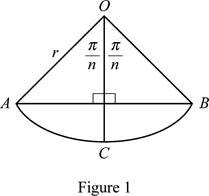# The expression of area of a polygon as 1 2 n r 2 sin ( 2 π n ) .### Single Variable Calculus: Concepts...

4th Edition
James Stewart
Publisher: Cengage Learning
ISBN: 9781337687805### Single Variable Calculus: Concepts...

4th Edition
James Stewart
Publisher: Cengage Learning
ISBN: 9781337687805

#### Solutions

Chapter 5.1, Problem 28E

(a)

To determine

## To show:The expression of area of a polygon as 12nr2sin(2πn).

Expert Solution

The area of a polygon An=12nr2sin(2πn) is proved.

### Explanation of Solution

Given information:

The area of the polygon is An (n-equal sides).

Polygon has n congruent triangles with central angle 2πn.

Calculation:

Procedure to draw one of the n congruent triangles in a circle is explained below:

• Let O be the center of the circle and AB be the one of the sides of the polygon.
• Draw the radius OC, so as to bisect the angle AOB. Also OC intersects AB at right angles and bisects AB.

Sketch one of the n congruent triangles in a circle with central angle 2πn as shown in Figure 1.Refer Figure 1.

The angle AOB is divided into 2 right angle triangles with legs of length 12(AB)=rsin(πn)rcos(πn)

Therefore, the triangle AOB has area of 212[rsin(πn)rcos(πn)] (1)

Rearrange Equation (1) as shown below.

AreaofΔAOB=[rsin(πn)rcos(πn)]=r2sin(πn)cos(πn)=12r2sin(2πn)

The area of the polygon with n equal sides (An) is expressed as follows:

An=n×AreaofΔAOB (2)

Substitute 12r2sin(2πn) for AreaofΔAOB in Equation (2).

An=n×12r2sin(2πn)=12nr2sin(2πn)

Therefore, the area of a polygon with n equal sides An is equal to 12nr2sin(2πn).

Therefore, The expression of area of a polygon as 12nr2sin(2πn).

(b)

To determine

### To show:The value of limn→∞An=πr2

Expert Solution

The value of limnAn=πr2 is proved.

### Explanation of Solution

Refer part (a).

The area of a polygon with n equal sides is An=12nr2sin(2πn).

Find the value of limnAn as shown below:

Substitute [12nr2sin(2πn)] for An in limnAn.

limnAn=limn12nr2sin(2πn)=limn12nr2sin(2πn)2πn2πn=limnsin(2πn)2πnπr2 (3)

Let θ=2πn.

As n, θ0, Substitute 2πn for θ in Equation (3).

limnsin(2πn)2πnπr2=limθ0sin(θ)θπr2=(1)πr2=πr2

Therefore, the value of limnAn is equal to πr2.

### Have a homework question?

Subscribe to bartleby learn! Ask subject matter experts 30 homework questions each month. Plus, you’ll have access to millions of step-by-step textbook answers!